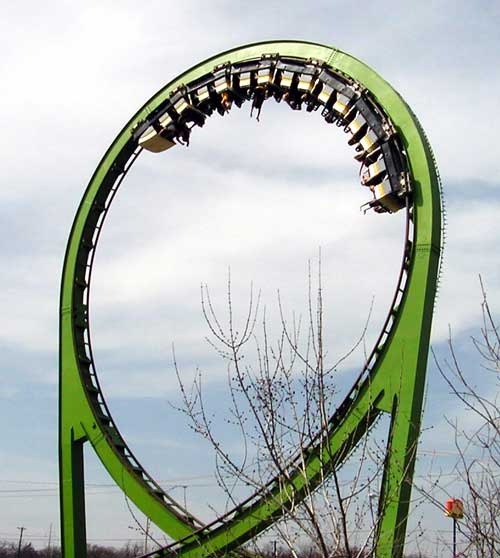# Is Gravity the only force doing work on a roller coaster cart?

• gazeem

#### gazeem

Homework Statement
Which of the following statements is true regarding a rider in a roller coaster cart moving with a constant speed through a loop?
Relevant Equations
(Choice A)
The sum of all the forces acting on the rider is zero.

(Choice B)
The rider is accelerating.

(Choice C)
Gravity is the only force doing work on the rider.

(Choice D)
There are two forces acting on the rider, but neither does any work on the rider.

--

The answer is Choice B, which makes sense; I'm just trying to figure out why C is not true.
Why I think gravity *is* the only force doing work on the rider:

1) The only forces acting on the rider are gravity and the normal force. Broken down into their component vectors, we have:
-> The component of the force of gravity moving parallel to the rider's direction of motion
-> The normal force, and the perpendicular component of gravity, acting in the perpendicular direction to the rider's direction of motion.

Thus, since Work is only the F applied in the direction of motion, gravity is the only force doing work on the rider.

But my guess is that perhaps something about the direction always changing means that the normal force is doing work on the rider by some definition? But that doesn't make sense to me because normal force will always be acting perpendicular to resulting displacement vector of its applied force.

Actually.. on second thought, perhaps normal force is in the same direction as the direction of motion, if you consider the direction of the displacement vector to be the direction of motion, and you break that vector into its vertical and horizontal components. So gravity and normal force would each be parallel to one of the constituent vectors of the displacement vector.

The answer is Choice A, which makes sense;
It doesn't.
If the net of all forces were zero it would move in a straight line at constant speed. We are told it is moving in a loop.

If a body moves at constant speed, what can you say about its KE? What does that tell you about the work done on it?

•etotheipi and gazeem
Oh I apologize, that's my bad I meant to refer to B) that it's accelerating.

as @haruspex noted, since we are given that the speed is constant, hence the Kinetic Energy of the rider is constant, we can infer from the work-energy theorem that the total Work done on the rider is zero. Which simply means that gravity isn't the only force doing work on the rider. No i don't think that the normal force does also work, i think that the roller wagon has some motor which is essentially doing the work to keep the speed constant.

•etotheipi and gazeem
Yes that makes sense, thank you. I was thrown off a bit by an explanation they gave that " In a vertical loop, there are 2 forces acting on the object: the normal force and gravity," but that is probably speaking generally and not comprehensively about this situation such that there must be some opposing force to the component of gravity parallel to the direction of motion.

•Delta2
i think that the roller wagon has some motor which is essentially doing the work to keep the speed constant.
No, I don't think they intended a motor to be considered.
We can rule out A and C, and B is certainly true, but I see no way to rule out D.
The question doesn't ask which of the choices is/are necessarily true. It could be executing a horizontal curve, so gravity and normal force act, neither doing work.

No, I don't think they intended a motor to be considered.
We can rule out A and C, and B is certainly true, but I see no way to rule out D.
The question doesn't ask which of the choices is/are necessarily true. It could be executing a horizontal curve, so gravity and normal force act, neither doing work.
I on the contrary , don't think that a horizontal curve ala Nascar is what they intended to be considered, When we talk about roller coaster we think about vertical loops that defy gravity.•